# Selina Solutions Concise Mathematics Class 6 Chapter 7: Number Line

Selina Solutions is a key source of study material for students. The solutions are well structured by experts with the purpose of helping students to solve each question with ease. Practicing the Selina Solutions Concise Mathematics on a regular basis increases students’ understanding capacity and problem solving skills. The diligent practice of Selina Solutions makes them understand basic concepts thoroughly. Selina Solutions are the best reliable resource among students to grasp the concepts easily as the answers provided are accurate with illustrations

A straight line with numbers placed at equal intervals along its length is called the Number Line. Our BYJU’S experts in the respective subject, designed the solutions to boost exam preparation among students. Accurate answers from Selina enables students to solve the complex problems easily. Download the PDF of Selina Solutions Concise Mathematics Class 6 Chapter 7 Number Line, from the links given below.

## Selina Solutions Concise Mathematics Class 6 Chapter 7: Number Line Download PDF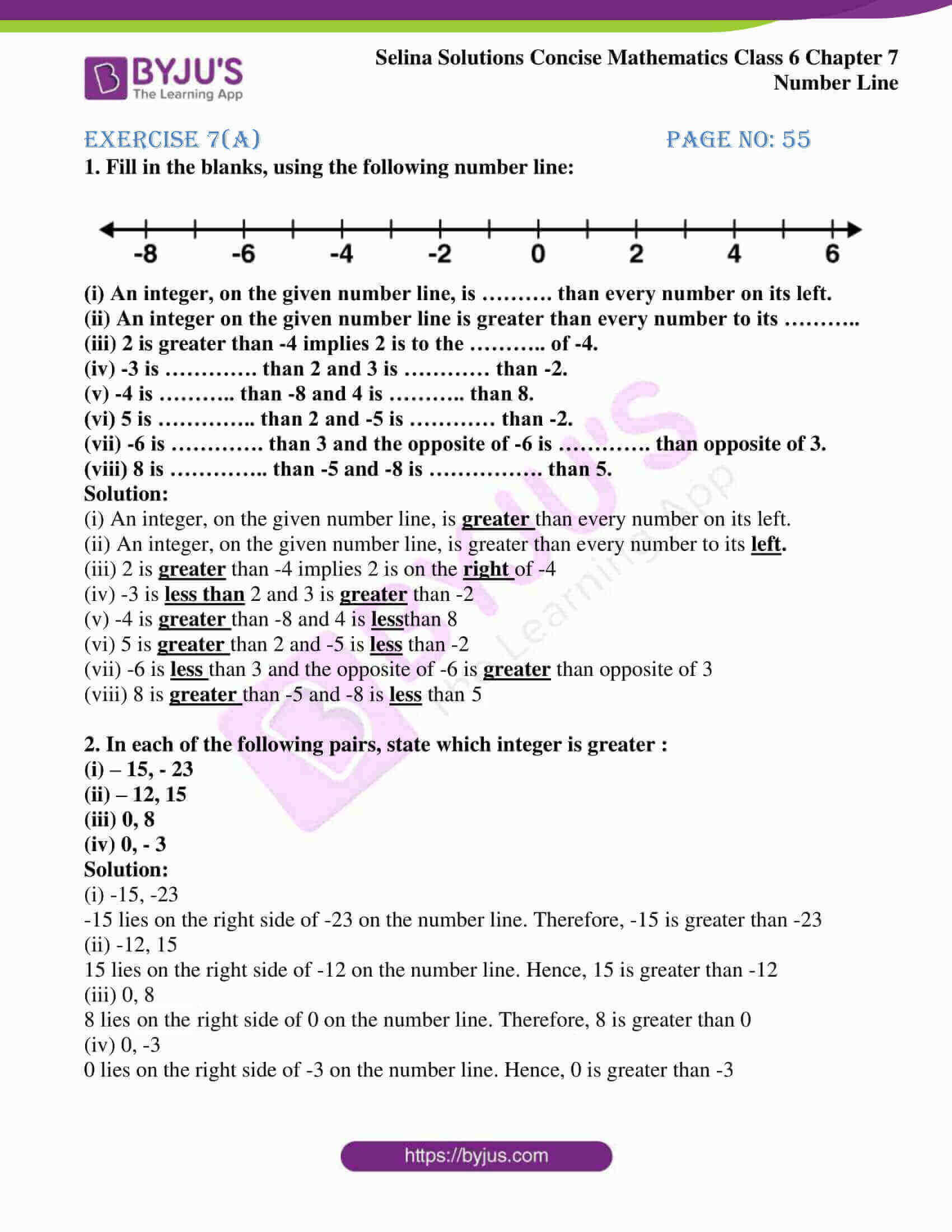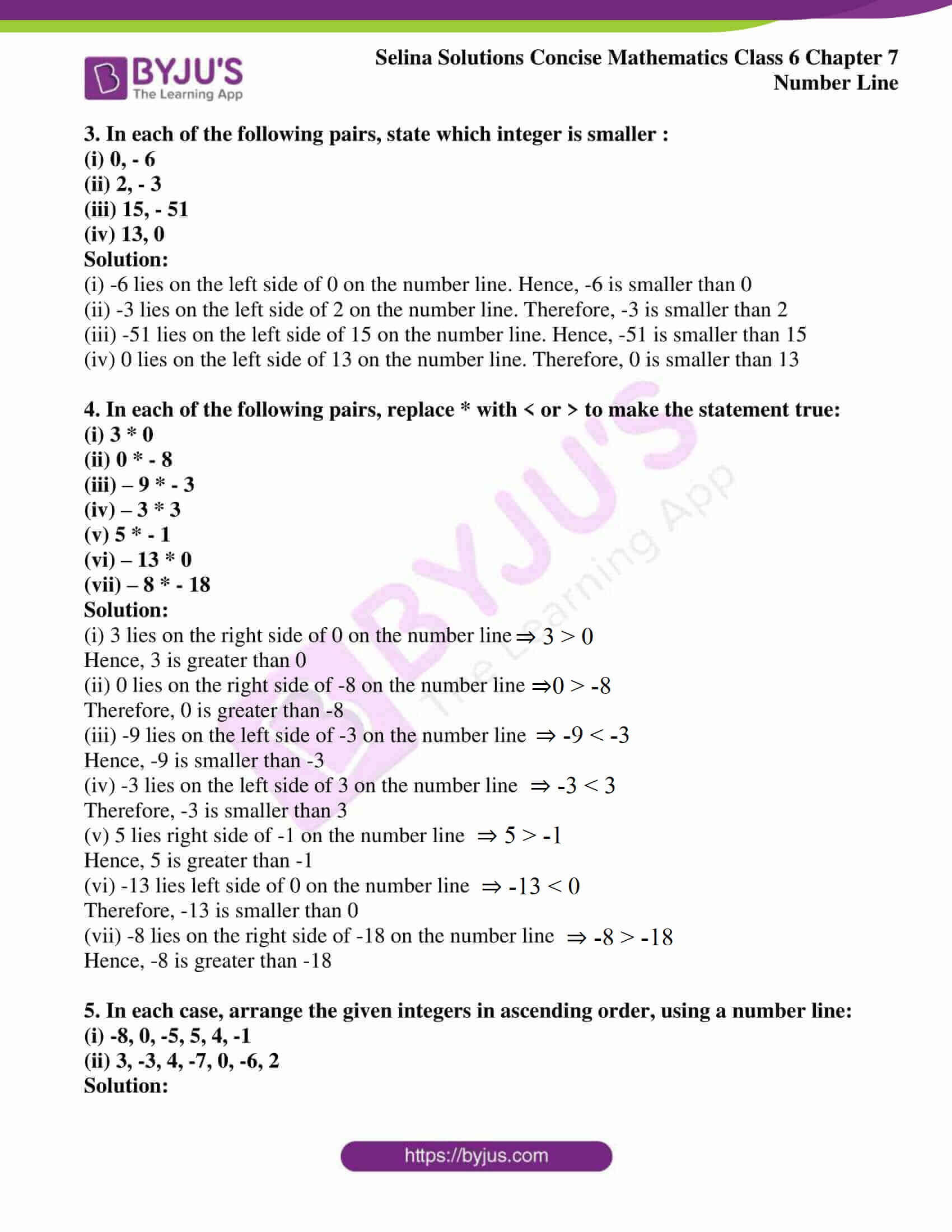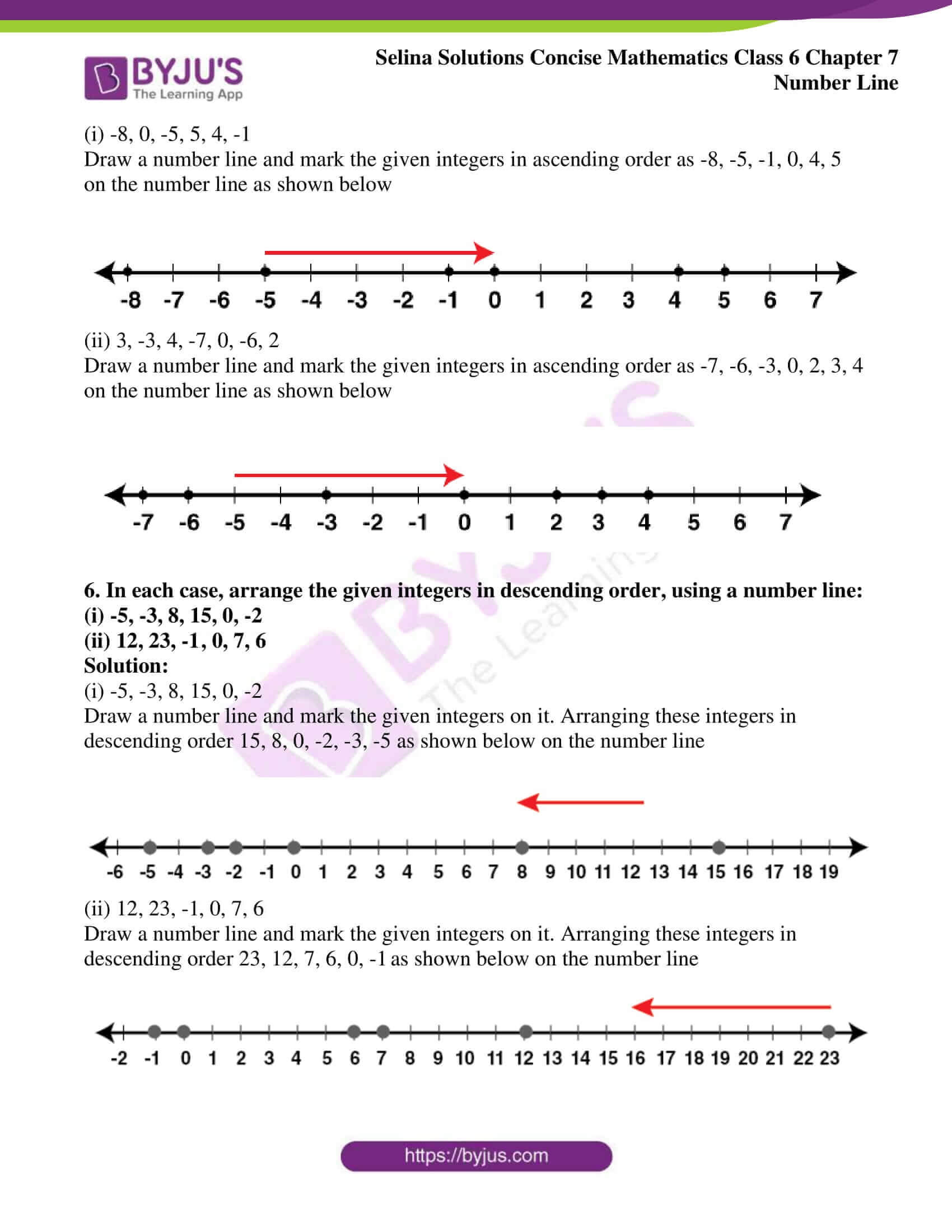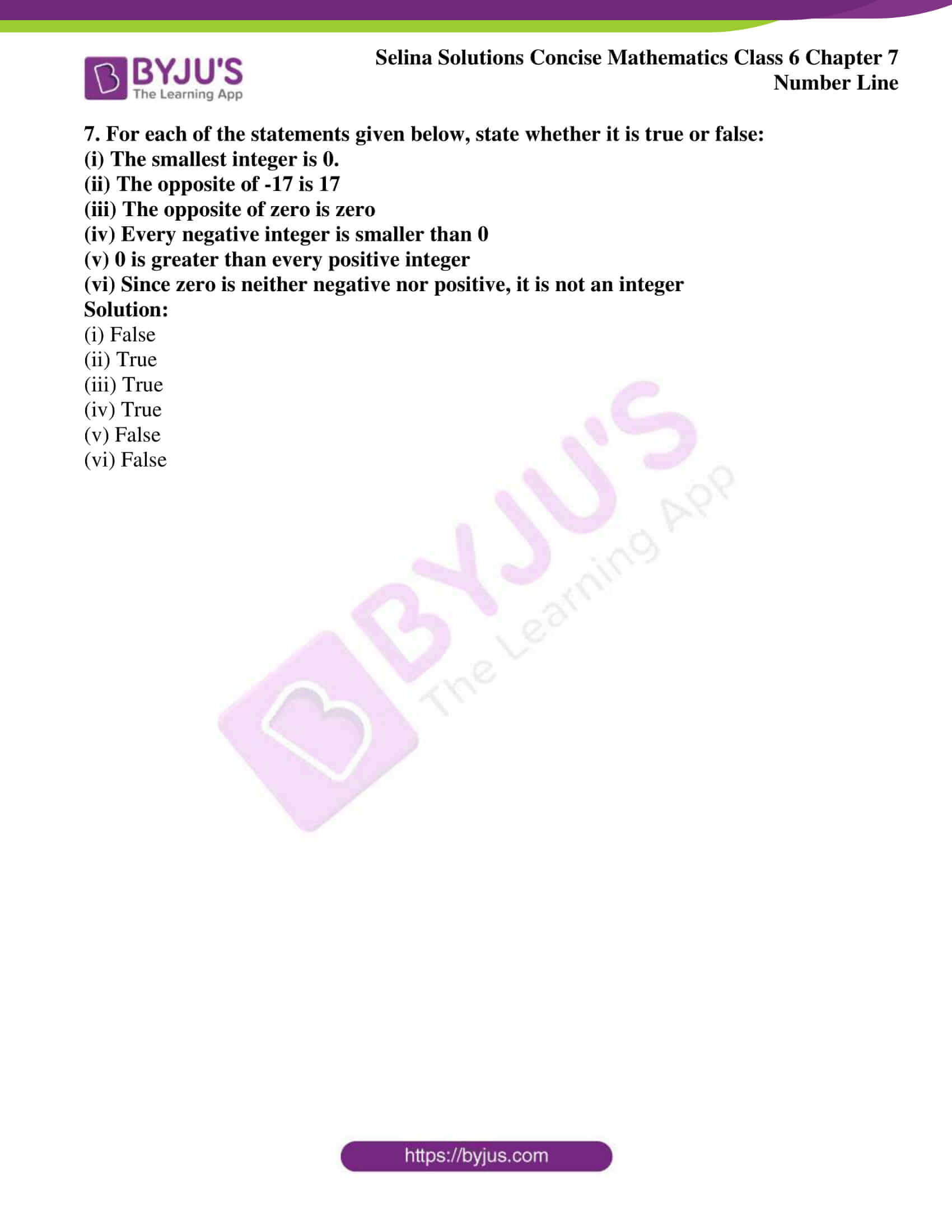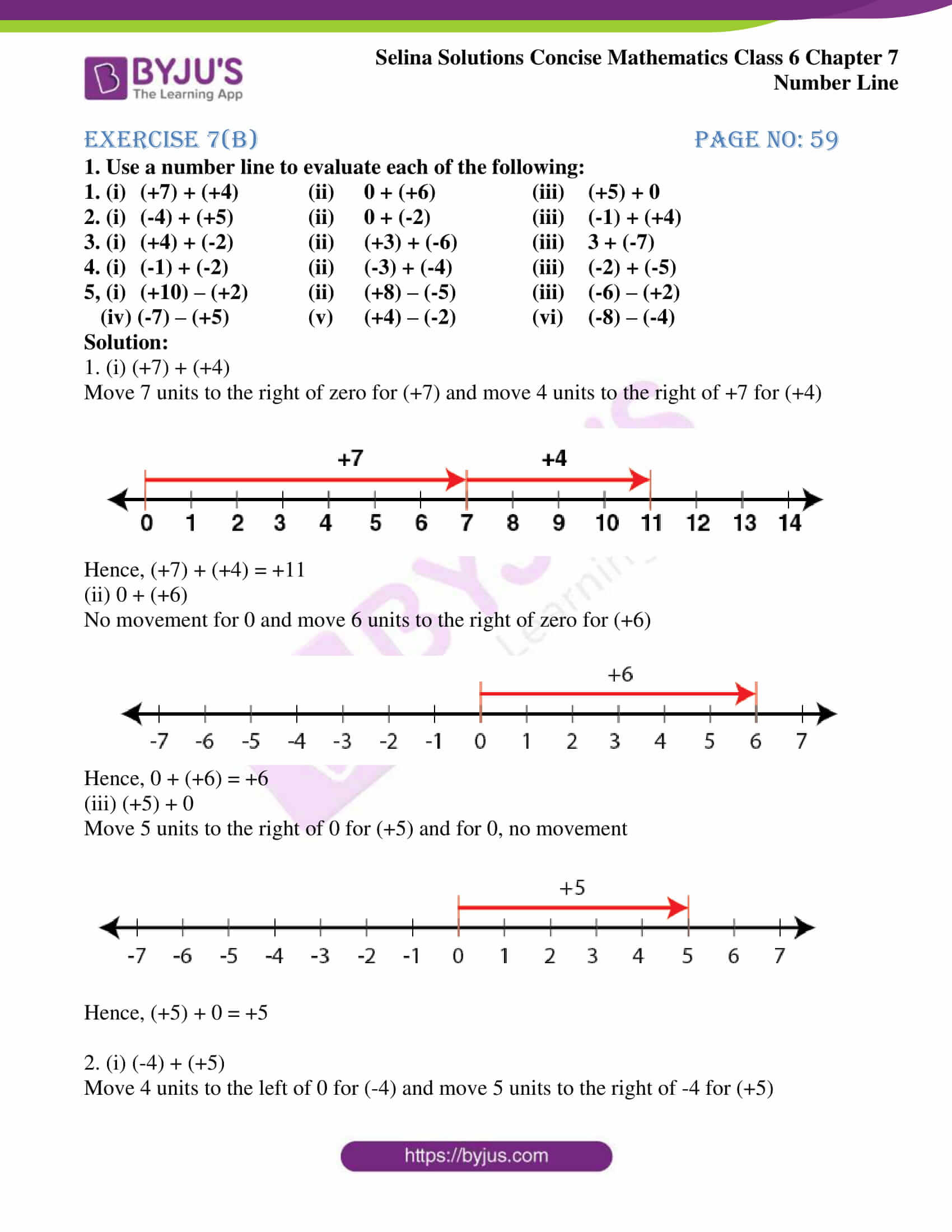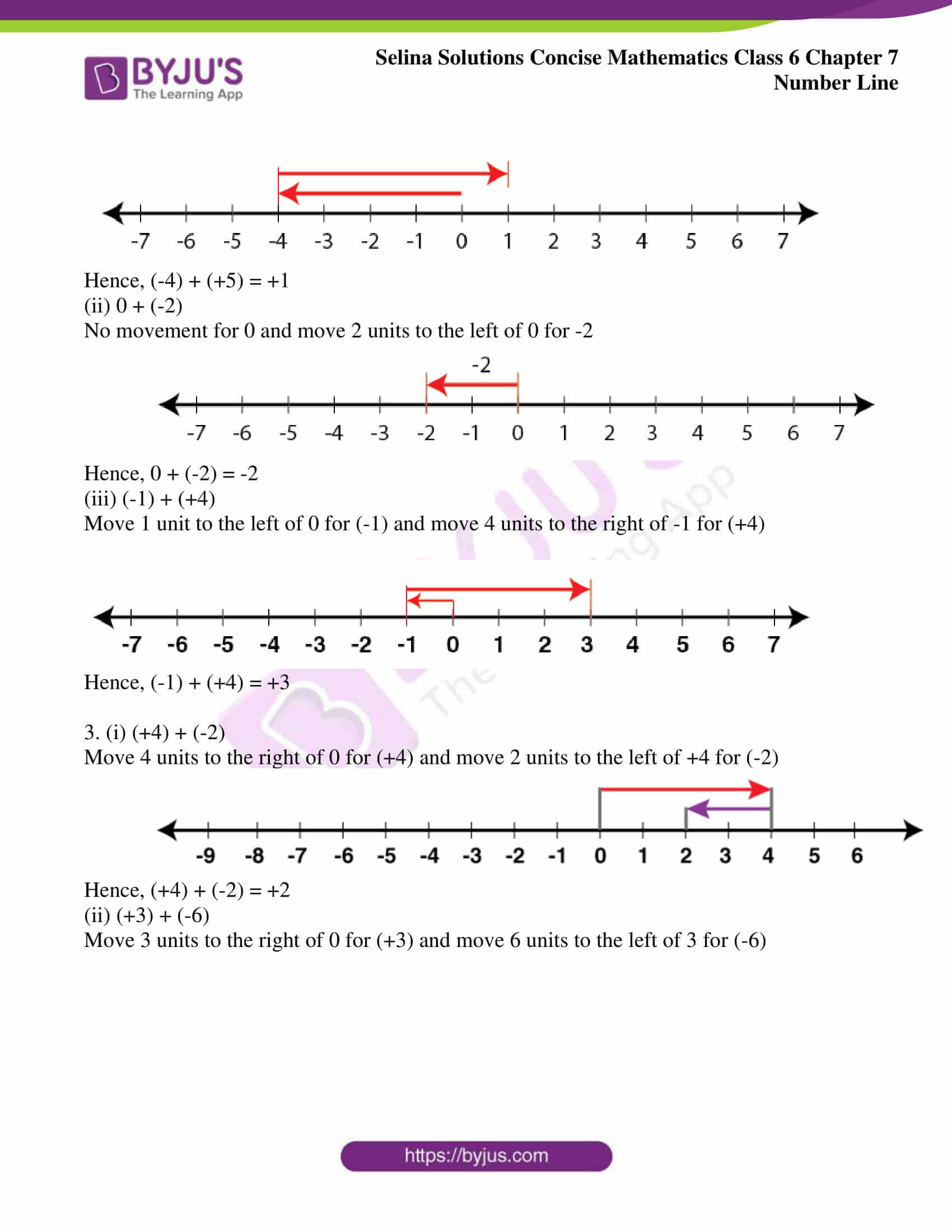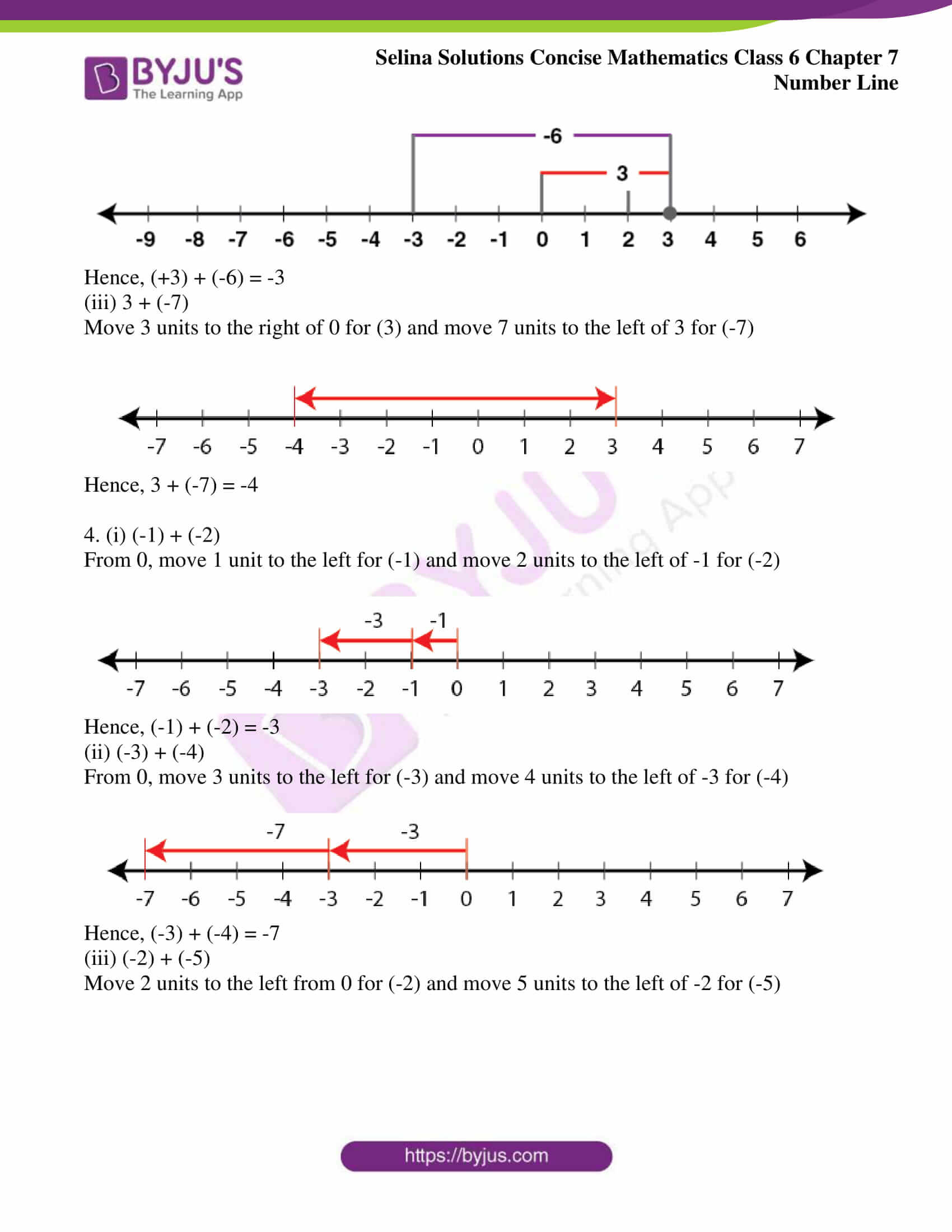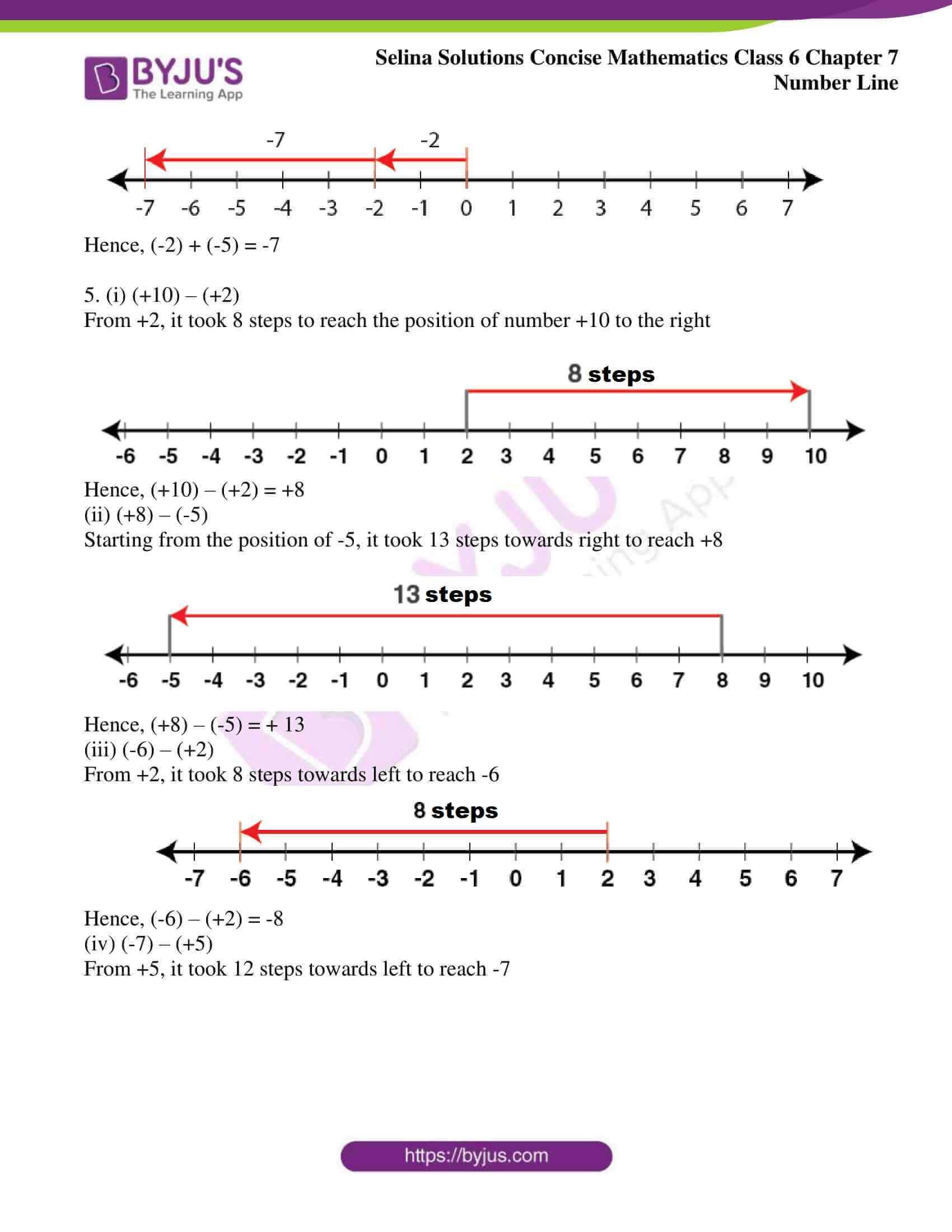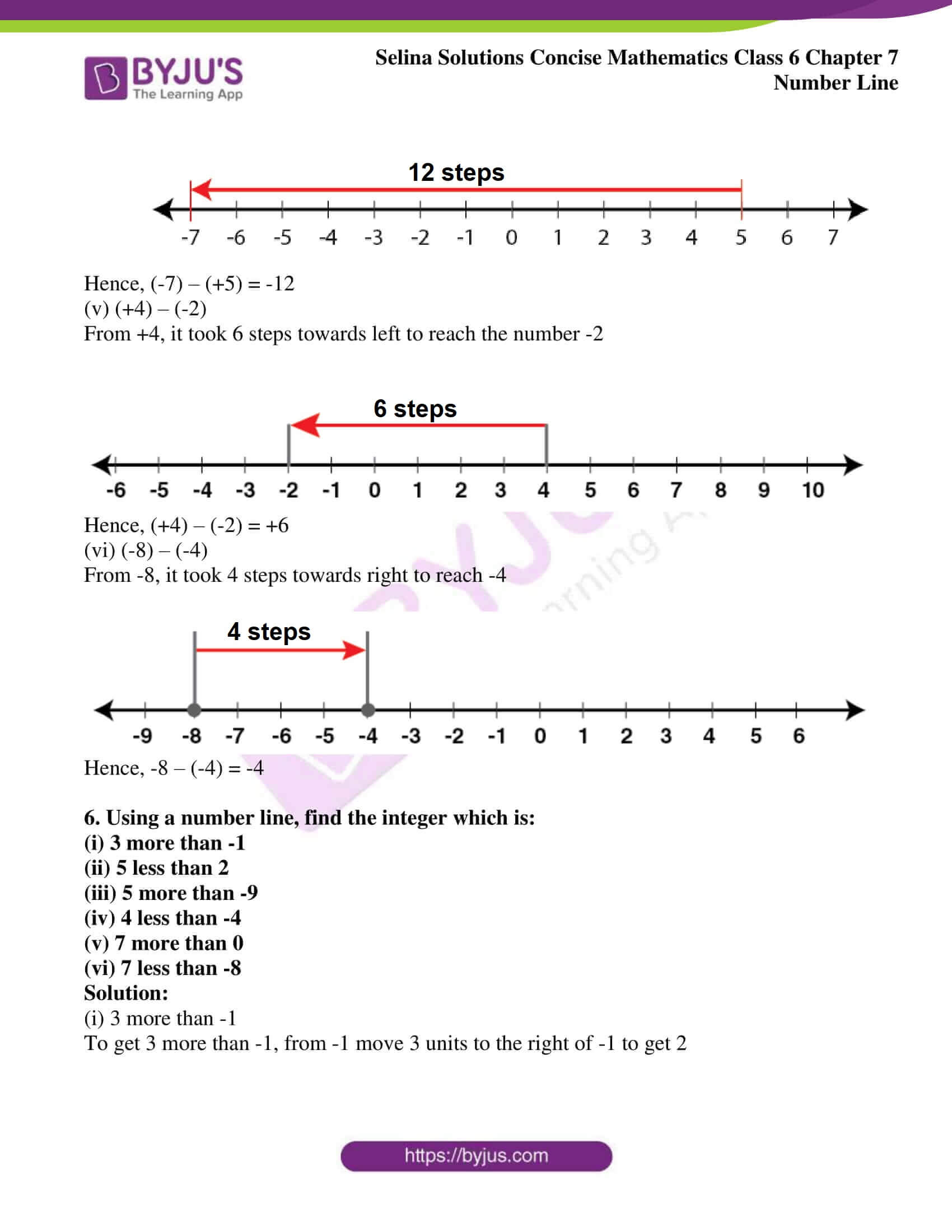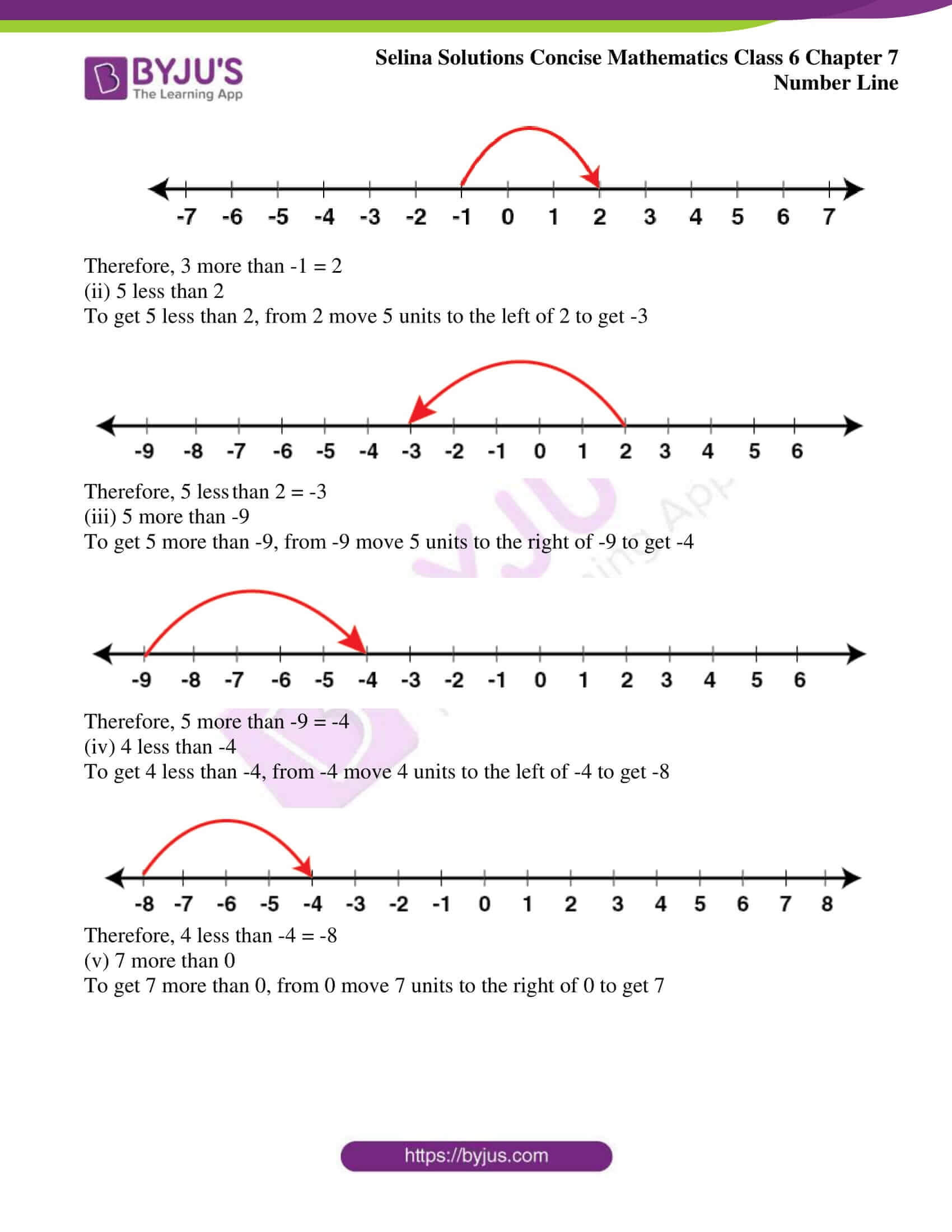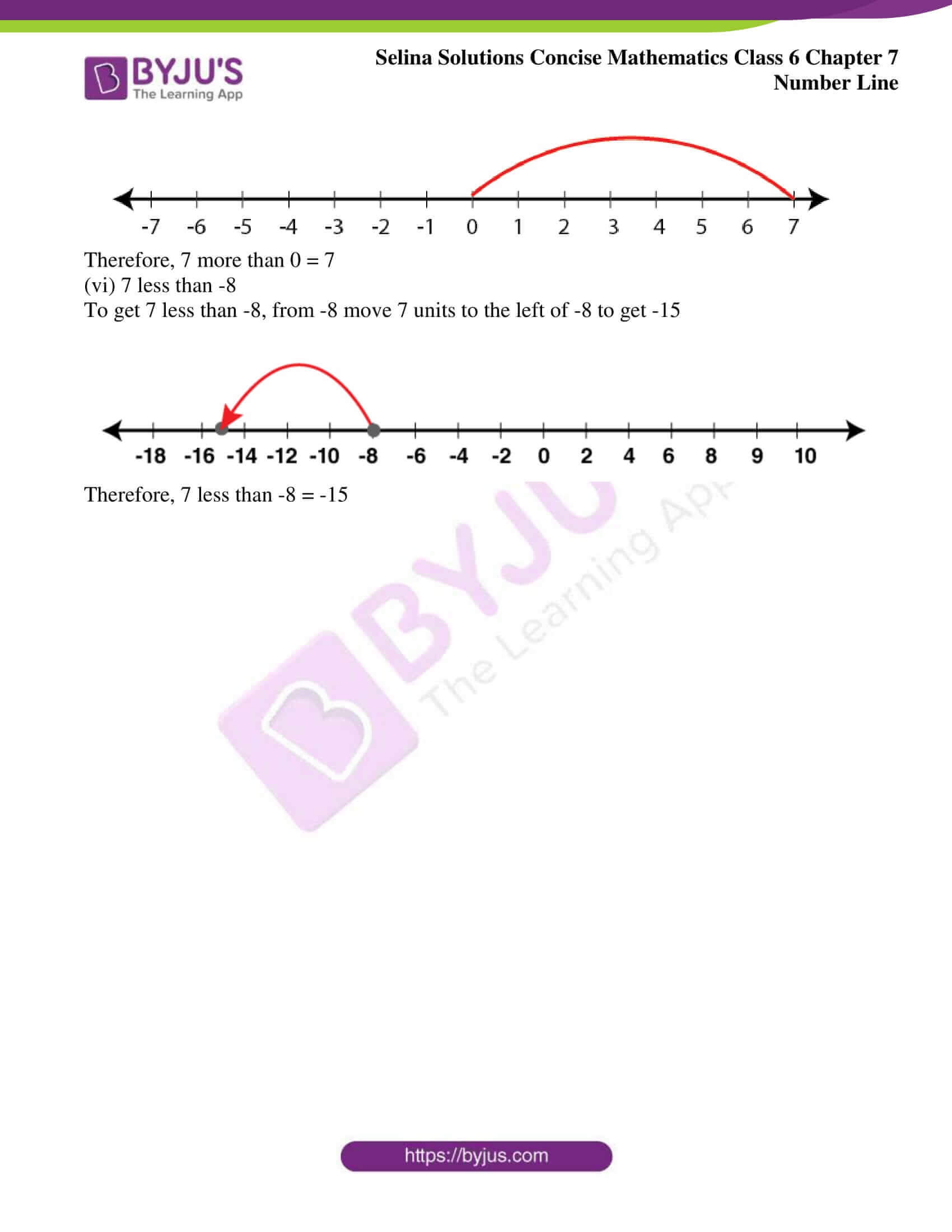### Exercises of Selina Solutions Concise Mathematics Class 6 Chapter 7: Number Line

Exercise 7(A) Solutions

Exercise 7(B) Solutions

## Access Selina Solutions Concise Mathematics Class 6 Chapter 7: Number Line

#### Exercise 7(A) page no: 55

1. Fill in the blanks, using the following number line: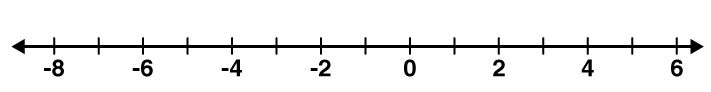(i) An integer, on the given number line, is ………. than every number on its left.

(ii) An integer on the given number line is greater than every number to its ………..

(iii) 2 is greater than -4 implies 2 is to the ……….. of -4.

(iv) -3 is …………. than 2 and 3 is ………… than -2.

(v) -4 is ……….. than -8 and 4 is ……….. than 8.

(vi) 5 is ………….. than 2 and -5 is ………… than -2.

(vii) -6 is …………. than 3 and the opposite of -6 is …………. than opposite of 3.

(viii) 8 is ………….. than -5 and -8 is ……………. than 5.

Solution:

(i) An integer, on the given number line, is greater than every number on its left.

(ii) An integer, on the given number line, is greater than every number to its left.

(iii) 2 is greater than -4 implies 2 is on the right of -4

(iv) -3 is less than 2 and 3 is greater than -2

(v) -4 is greater than -8 and 4 is lessthan 8

(vi) 5 is greater than 2 and -5 is less than -2

(vii) -6 is less than 3 and the opposite of -6 is greater than opposite of 3

(viii) 8 is greater than -5 and -8 is less than 5

2. In each of the following pairs, state which integer is greater :

(i) – 15, – 23

(ii) – 12, 15

(iii) 0, 8

(iv) 0, – 3

Solution:

(i) -15, -23

-15 lies on the right side of -23 on the number line. Therefore, -15 is greater than -23

(ii) -12, 15

15 lies on the right side of -12 on the number line. Hence, 15 is greater than -12

(iii) 0, 8

8 lies on the right side of 0 on the number line. Therefore, 8 is greater than 0

(iv) 0, -3

0 lies on the right side of -3 on the number line. Hence, 0 is greater than -3

3. In each of the following pairs, state which integer is smaller :

(i) 0, – 6

(ii) 2, – 3

(iii) 15, – 51

(iv) 13, 0

Solution:

(i) -6 lies on the left side of 0 on the number line. Hence, -6 is smaller than 0

(ii) -3 lies on the left side of 2 on the number line. Therefore, -3 is smaller than 2

(iii) -51 lies on the left side of 15 on the number line. Hence, -51 is smaller than 15

(iv) 0 lies on the left side of 13 on the number line. Therefore, 0 is smaller than 13

4. In each of the following pairs, replace * with < or > to make the statement true:

(i) 3 * 0

(ii) 0 * – 8

(iii) – 9 * – 3

(iv) – 3 * 3

(v) 5 * – 1

(vi) – 13 * 0

(vii) – 8 * – 18

Solution:

(i) 3 lies on the right side of 0 on the number line ⇒ 3 > 0

Hence, 3 is greater than 0

(ii) 0 lies on the right side of -8 on the number line ⇒ 0 > -8

Therefore, 0 is greater than -8

(iii) -9 lies on the left side of -3 on the number line ⇒ -9 < -3

Hence, -9 is smaller than -3

(iv) -3 lies on the left side of 3 on the number line ⇒ -3 < 3

Therefore, -3 is smaller than 3

(v) 5 lies right side of -1 on the number line ⇒ 5 > -1

Hence, 5 is greater than -1

(vi) -13 lies left side of 0 on the number line ⇒ -13 < 0

Therefore, -13 is smaller than 0

(vii) -8 lies on the right side of -18 on the number line ⇒ -8 > -18

Hence, -8 is greater than -18

5. In each case, arrange the given integers in ascending order, using a number line:

(i) -8, 0, -5, 5, 4, -1

(ii) 3, -3, 4, -7, 0, -6, 2

Solution:

(i) -8, 0, -5, 5, 4, -1

Draw a number line and mark the given integers in ascending order as -8, -5, -1, 0, 4, 5 on the number line as shown below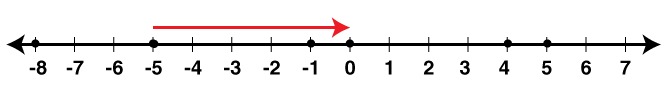(ii) 3, -3, 4, -7, 0, -6, 2

Draw a number line and mark the given integers in ascending order as -7, -6, -3, 0, 2, 3, 4 on the number line as shown below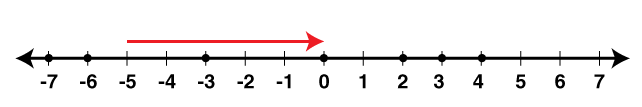6. In each case, arrange the given integers in descending order, using a number line:

(i) -5, -3, 8, 15, 0, -2

(ii) 12, 23, -1, 0, 7, 6

Solution:

(i) -5, -3, 8, 15, 0, -2

Draw a number line and mark the given integers on it. Arranging these integers in descending order 15, 8, 0, -2, -3, -5 as shown below on the number line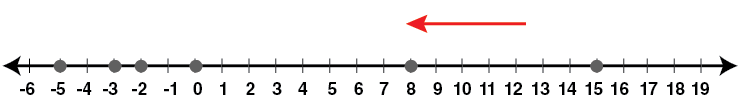(ii) 12, 23, -1, 0, 7, 6

Draw a number line and mark the given integers on it. Arranging these integers in descending order 23, 12, 7, 6, 0, -1 as shown below on the number line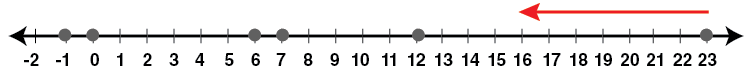7. For each of the statements given below, state whether it is true or false:

(i) The smallest integer is 0.

(ii) The opposite of -17 is 17

(iii) The opposite of zero is zero

(iv) Every negative integer is smaller than 0

(v) 0 is greater than every positive integer

(vi) Since zero is neither negative nor positive, it is not an integer

Solution:

(i) False

(ii) True

(iii) True

(iv) True

(v) False

(vi) False

#### Exercise 7(B) page no: 59

1. Use a number line to evaluate each of the following:

1. (i) (+7) + (+4) (ii) 0 + (+6) (iii) (+5) + 0

2. (i) (-4) + (+5) (ii) 0 + (-2) (iii) (-1) + (+4)

3. (i) (+4) + (-2) (ii) (+3) + (-6) (iii) 3 + (-7)

4. (i) (-1) + (-2) (ii) (-3) + (-4) (iii) (-2) + (-5)

5, (i) (+10) – (+2) (ii) (+8) – (-5) (iii) (-6) – (+2)

(iv) (-7) – (+5) (v) (+4) – (-2) (vi) (-8) – (-4)

Solution:

1. (i) (+7) + (+4)

Move 7 units to the right of zero for (+7) and move 4 units to the right of +7 for (+4)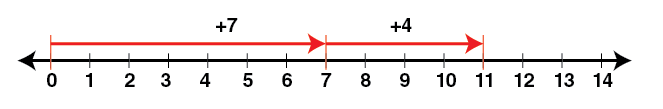Hence, (+7) + (+4) = +11

(ii) 0 + (+6)

No movement for 0 and move 6 units to the right of zero for (+6)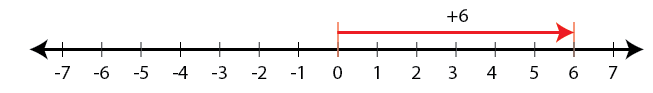Hence, 0 + (+6) = +6

(iii) (+5) + 0

Move 5 units to the right of 0 for (+5) and for 0, no movement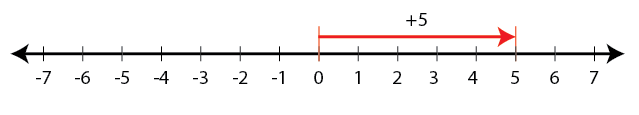Hence, (+5) + 0 = +5

2. (i) (-4) + (+5)

Move 4 units to the left of 0 for (-4) and move 5 units to the right of -4 for (+5)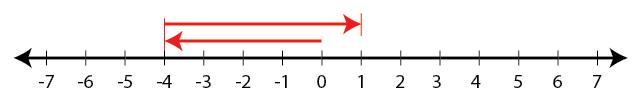Hence, (-4) + (+5) = +1

(ii) 0 + (-2)

No movement for 0 and move 2 units to the left of 0 for -2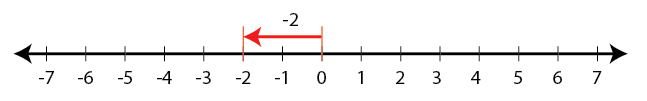Hence, 0 + (-2) = -2

(iii) (-1) + (+4)

Move 1 unit to the left of 0 for (-1) and move 4 units to the right of -1 for (+4)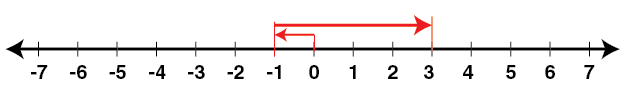Hence, (-1) + (+4) = +3

3. (i) (+4) + (-2)

Move 4 units to the right of 0 for (+4) and move 2 units to the left of +4 for (-2)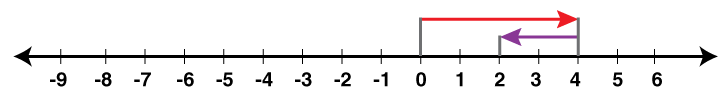Hence, (+4) + (-2) = +2

(ii) (+3) + (-6)

Move 3 units to the right of 0 for (+3) and move 6 units to the left of 3 for (-6)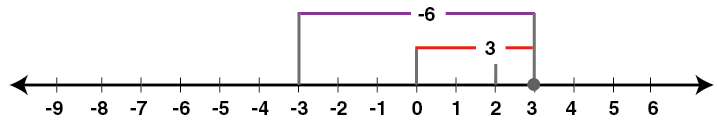Hence, (+3) + (-6) = -3

(iii) 3 + (-7)

Move 3 units to the right of 0 for (3) and move 7 units to the left of 3 for (-7)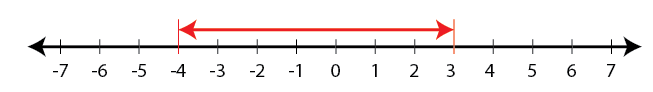Hence, 3 + (-7) = -4

4. (i) (-1) + (-2)

From 0, move 1 unit to the left for (-1) and move 2 units to the left of -1 for (-2)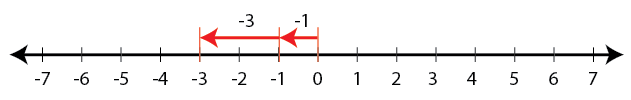Hence, (-1) + (-2) = -3

(ii) (-3) + (-4)

From 0, move 3 units to the left for (-3) and move 4 units to the left of -3 for (-4)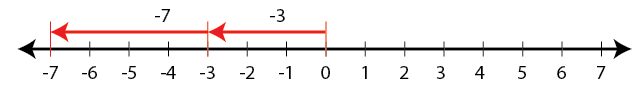Hence, (-3) + (-4) = -7

(iii) (-2) + (-5)

Move 2 units to the left from 0 for (-2) and move 5 units to the left of -2 for (-5)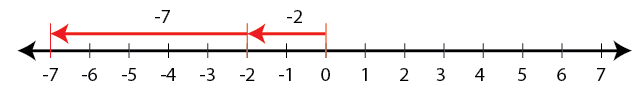Hence, (-2) + (-5) = -7

5. (i) (+10) – (+2)

From +2, it took 8 steps to reach the position of number +10 to the right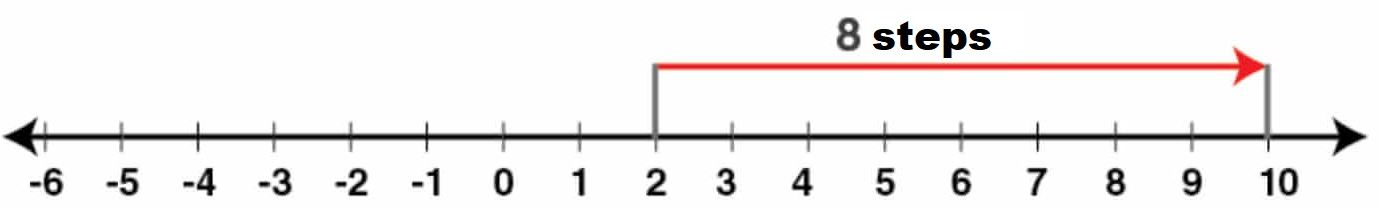Hence, (+10) – (+2) = +8

(ii) (+8) – (-5)

Starting from the position of -5, it took 13 steps towards right to reach +8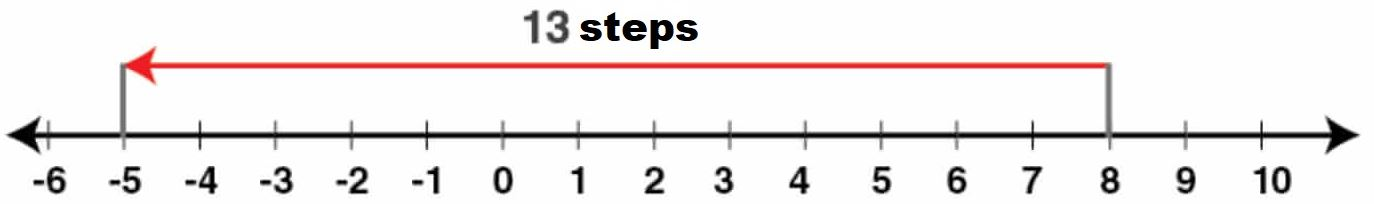Hence, (+8) – (-5) = + 13

(iii) (-6) – (+2)

From +2, it took 8 steps towards left to reach -6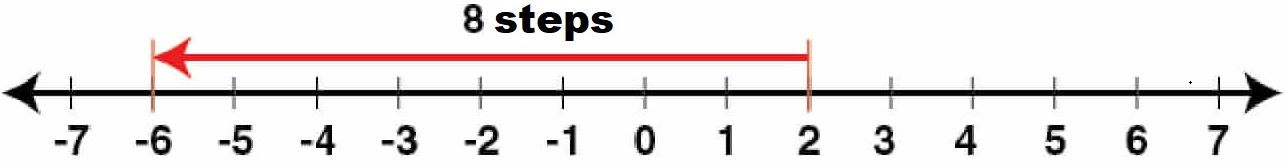Hence, (-6) – (+2) = -8

(iv) (-7) – (+5)

From +5, it took 12 steps towards left to reach -7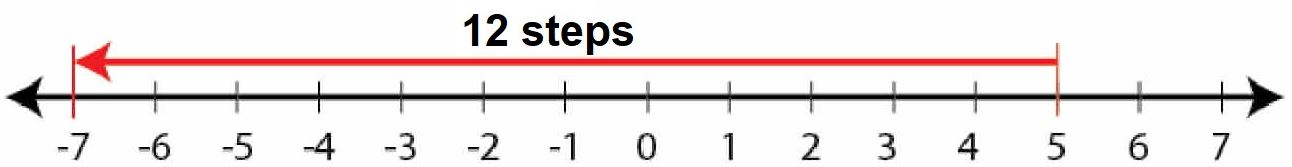Hence, (-7) – (+5) = -12

(v) (+4) – (-2)

From +4, it took 6 steps towards left to reach the number -2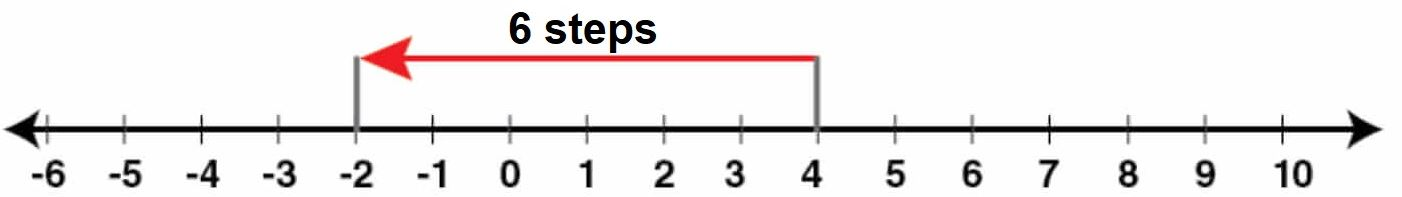Hence, (+4) – (-2) = +6

(vi) (-8) – (-4)

From -8, it took 4 steps towards right to reach -4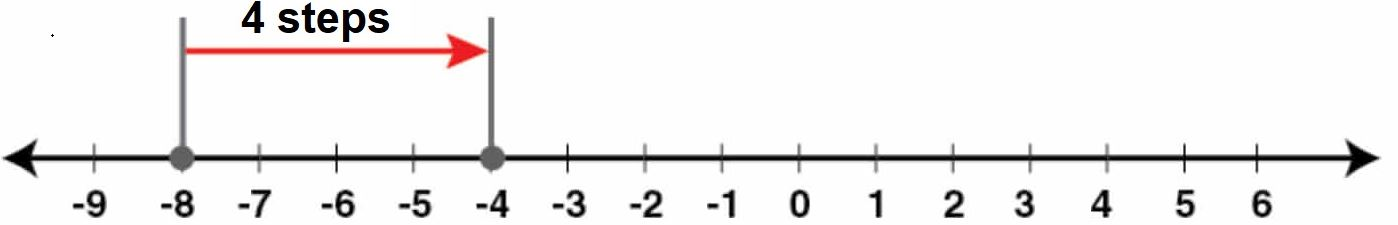Hence, -8 – (-4) = -4

6. Using a number line, find the integer which is:

(i) 3 more than -1

(ii) 5 less than 2

(iii) 5 more than -9

(iv) 4 less than -4

(v) 7 more than 0

(vi) 7 less than -8

Solution:

(i) 3 more than -1

To get 3 more than -1, from -1 move 3 units to the right of -1 to get 2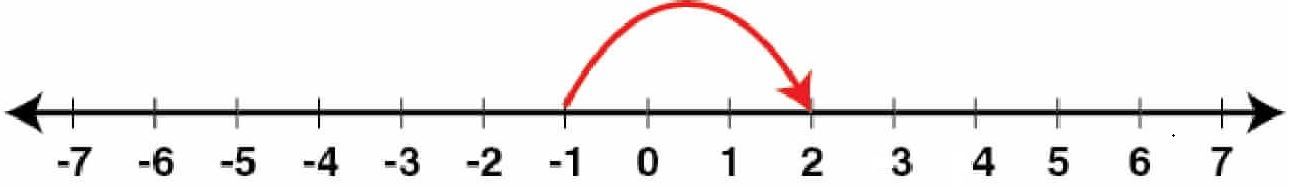Therefore, 3 more than -1 = 2

(ii) 5 less than 2

To get 5 less than 2, from 2 move 5 units to the left of 2 to get -3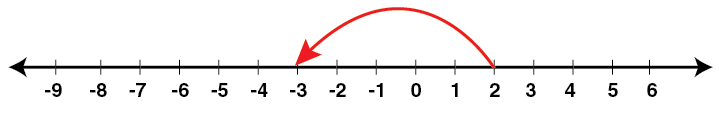Therefore, 5 less than 2 = -3

(iii) 5 more than -9

To get 5 more than -9, from -9 move 5 units to the right of -9 to get -4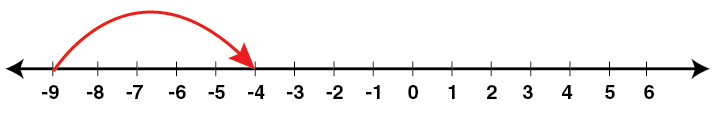Therefore, 5 more than -9 = -4

(iv) 4 less than -4

To get 4 less than -4, from -4 move 4 units to the left of -4 to get -8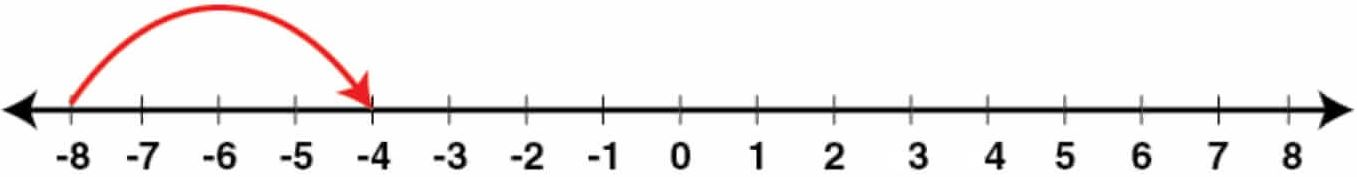Therefore, 4 less than -4 = -8

(v) 7 more than 0

To get 7 more than 0, from 0 move 7 units to the right of 0 to get 7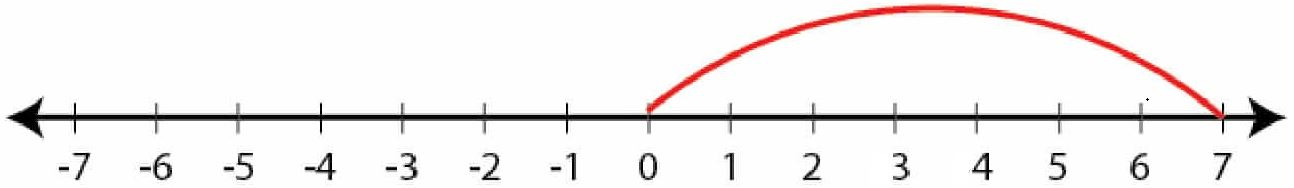Therefore, 7 more than 0 = 7

(vi) 7 less than -8

To get 7 less than -8, from -8 move 7 units to the left of -8 to get -15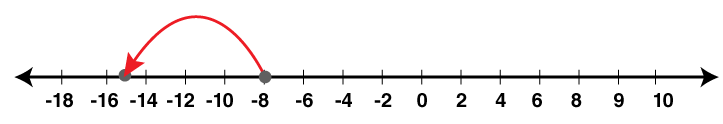Therefore, 7 less than -8 = -15